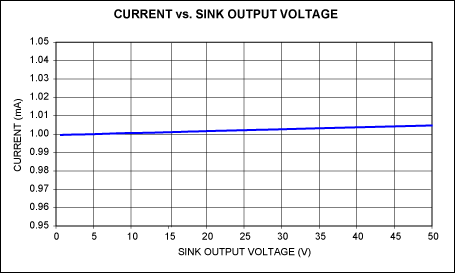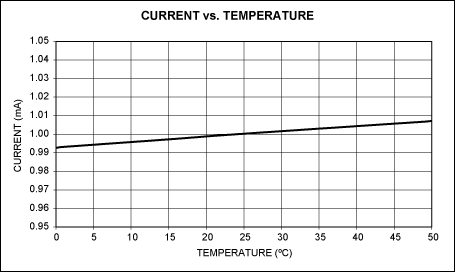# Precision Constant-Current Sink Provides Wide, Close-to-the-Rail Compliance

### Abstract

This precision constant-current sink produces a current of 1mA with a compliance range of 0.7V to 50V, and a dynamic output impedance of 106 ohms. It is stable with any load type (resistive or reactive), and stable over the allowed ranges of temperature, power-supply voltage, and compliance voltage (upper and lower limits).

A similar version of this article appeared in the February 2009 issue of Portable Design magazine.

A precision current sink can be used to generate a voltage bias for sensors, amplifiers, and other analog circuits. They can also generate a reference voltage (across a resistor in series with its output) referred to voltage levels that are variable or well above the common equipotential. Such current sinks consist of a voltage reference, an error amplifier, a current-sense resistor, and an output device—usually one capable of handling a useful amount of power, and rated for voltages at the expected upper limit of the output-compliance range.

Desirable characteristics for this type of circuit block include a very large dynamic output impedance, stability with any load type (resistive or reactive), and stability over the allowed ranges of temperature, power-supply voltage, and compliance voltage (upper and lower limits).

The circuit of Figure 1a, with component values as shown, produces a current of 1mA with a compliance range of 0.7V to 50V, and a dynamic output impedance of 106 ohms. The circuit of Figure 1b can do the same, with a compliance range that extends down to 0.25V, but it requires an extra, separate reference voltage.The reference/amplifier function is implemented with a shunt regulator (MAX8515), and the power output device (BSS123) is a small n-channel MOSFET with maximum VDS of 100V (VDS is the current sink's higher compliance limit). The current sample is obtained from a resistor (RSOURCE) in series with the BSS123 source. You can adjust this current by changing the resistor value according to the following equation:

Circuit of Figure 1a: ISINK = 0.6V/RSOURCE.

Circuit of Figure 1b: ISINK = 0.091V/RSOURCE.

Other performance includes the stability of sink current with output voltage (Figure 2), and the variation of sink current with temperature over the range 0°C to +50°C (±0.75%, Figure 3).